# Convolutional Neural Networks (CNN)

Convolutional Neural Networks (CNNs) applied to text for natural language processing (NLP) tasks.Goku Mohandas
· · ·
Repository · Notebook

📬  Receive new lessons straight to your inbox (once a month) and join 40K+ developers in learning how to responsibly deliver value with ML.

## Overview

At the core of CNNs are filters (aka weights, kernels, etc.) which convolve (slide) across our input to extract relevant features. The filters are initialized randomly but learn to act as feature extractors via parameter sharing.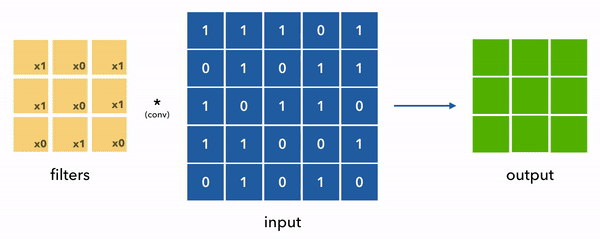• Objective:
• Extract meaningful spatial substructure from encoded data.
• Small number of weights (shared)
• Parallelizable
• Detects spatial substrcutures (feature extractors)
• Interpretability via filters
• Can be used for processing in images, text, time-series, etc.
• Many hyperparameters (kernel size, strides, etc.) to tune.
• Miscellaneous:
• Lot's of deep CNN architectures constantly updated for SOTA performance.
• Very popular feature extractor that acts as a foundation for many architectures.

## Set up

Let's set our seed and device.

 1 2 3 4 5 import numpy as np import pandas as pd import random import torch import torch.nn as nn 
 1 SEED = 1234 
 1 2 3 4 5 6 7 def set_seeds(seed=1234): """Set seeds for reproducibility.""" np.random.seed(seed) random.seed(seed) torch.manual_seed(seed) torch.cuda.manual_seed(seed) torch.cuda.manual_seed_all(seed) # multi-GPU 
 1 2 # Set seeds for reproducibility set_seeds(seed=SEED) 
 1 2 3 4 5 6 7 8 # Set device cuda = True device = torch.device("cuda" if ( torch.cuda.is_available() and cuda) else "cpu") torch.set_default_tensor_type("torch.FloatTensor") if device.type == "cuda": torch.set_default_tensor_type("torch.cuda.FloatTensor") print (device) 

cuda


We will download the AG News dataset, which consists of 120K text samples from 4 unique classes (Business, Sci/Tech, Sports, World)

 1 2 3 4 5 # Load data url = "https://raw.githubusercontent.com/GokuMohandas/Made-With-ML/main/datasets/news.csv" df = pd.read_csv(url, header=0) # load df = df.sample(frac=1).reset_index(drop=True) # shuffle df.head() 

title category
0 Sharon Accepts Plan to Reduce Gaza Army Operation... World
1 Internet Key Battleground in Wildlife Crime Fight Sci/Tech
2 July Durable Good Orders Rise 1.7 Percent Business
3 Growing Signs of a Slowing on Wall Street Business
4 The New Faces of Reality TV World

### Preprocessing

We're going to clean up our input data first by doing operations such as lower text, removing stop (filler) words, filters using regular expressions, etc.

 1 2 3 4 import nltk from nltk.corpus import stopwords from nltk.stem import PorterStemmer import re 
 1 2 3 4 nltk.download("stopwords") STOPWORDS = stopwords.words("english") print (STOPWORDS[:5]) porter = PorterStemmer() 

[nltk_data] Downloading package stopwords to /root/nltk_data...
[nltk_data]   Package stopwords is already up-to-date!
['i', 'me', 'my', 'myself', 'we']


  1 2 3 4 5 6 7 8 9 10 11 12 13 14 15 16 17 18 19 def preprocess(text, stopwords=STOPWORDS): """Conditional preprocessing on our text unique to our task.""" # Lower text = text.lower() # Remove stopwords pattern = re.compile(r"\b(" + r"|".join(stopwords) + r")\b\s*") text = pattern.sub("", text) # Remove words in parenthesis text = re.sub(r"$$[^)]*$$", "", text) # Spacing and filters text = re.sub(r"([-;;.,!?<=>])", r" \1 ", text) # separate punctuation tied to words text = re.sub("[^A-Za-z0-9]+", " ", text) # remove non alphanumeric chars text = re.sub(" +", " ", text) # remove multiple spaces text = text.strip() return text 
 1 2 3 # Sample text = "Great week for the NYSE!" preprocess(text=text) 

great week nyse

 1 2 3 4 # Apply to dataframe preprocessed_df = df.copy() preprocessed_df.title = preprocessed_df.title.apply(preprocess) print (f"{df.title.values}\n\n{preprocessed_df.title.values}") 
Sharon Accepts Plan to Reduce Gaza Army Operation, Haaretz Says

sharon accepts plan reduce gaza army operation haaretz says


### Split data

 1 2 import collections from sklearn.model_selection import train_test_split 
 1 2 3 TRAIN_SIZE = 0.7 VAL_SIZE = 0.15 TEST_SIZE = 0.15 
 1 2 3 4 5 def train_val_test_split(X, y, train_size): """Split dataset into data splits.""" X_train, X_, y_train, y_ = train_test_split(X, y, train_size=TRAIN_SIZE, stratify=y) X_val, X_test, y_val, y_test = train_test_split(X_, y_, train_size=0.5, stratify=y_) return X_train, X_val, X_test, y_train, y_val, y_test 
 1 2 3 # Data X = preprocessed_df["title"].values y = preprocessed_df["category"].values 
 1 2 3 4 5 6 7 # Create data splits X_train, X_val, X_test, y_train, y_val, y_test = train_val_test_split( X=X, y=y, train_size=TRAIN_SIZE) print (f"X_train: {X_train.shape}, y_train: {y_train.shape}") print (f"X_val: {X_val.shape}, y_val: {y_val.shape}") print (f"X_test: {X_test.shape}, y_test: {y_test.shape}") print (f"Sample point: {X_train} → {y_train}") 

X_train: (84000,), y_train: (84000,)
X_val: (18000,), y_val: (18000,)
X_test: (18000,), y_test: (18000,)
Sample point: china battles north korea nuclear talks → World


### Label encoding

Next we'll define a LabelEncoder to encode our text labels into unique indices

 1 import itertools 
  1 2 3 4 5 6 7 8 9 10 11 12 13 14 15 16 17 18 19 20 21 22 23 24 25 26 27 28 29 30 31 32 33 34 35 36 37 38 39 40 41 42 43 class LabelEncoder(object): """Label encoder for tag labels.""" def __init__(self, class_to_index={}): self.class_to_index = class_to_index or {} # mutable defaults ;) self.index_to_class = {v: k for k, v in self.class_to_index.items()} self.classes = list(self.class_to_index.keys()) def __len__(self): return len(self.class_to_index) def __str__(self): return f"" def fit(self, y): classes = np.unique(y) for i, class_ in enumerate(classes): self.class_to_index[class_] = i self.index_to_class = {v: k for k, v in self.class_to_index.items()} self.classes = list(self.class_to_index.keys()) return self def encode(self, y): encoded = np.zeros((len(y)), dtype=int) for i, item in enumerate(y): encoded[i] = self.class_to_index[item] return encoded def decode(self, y): classes = [] for i, item in enumerate(y): classes.append(self.index_to_class[item]) return classes def save(self, fp): with open(fp, "w") as fp: contents = {'class_to_index': self.class_to_index} json.dump(contents, fp, indent=4, sort_keys=False) @classmethod def load(cls, fp): with open(fp, "r") as fp: kwargs = json.load(fp=fp) return cls(**kwargs) 
 1 2 3 4 5 # Encode label_encoder = LabelEncoder() label_encoder.fit(y_train) NUM_CLASSES = len(label_encoder) label_encoder.class_to_index 

{'Business': 0, 'Sci/Tech': 1, 'Sports': 2, 'World': 3}

 1 2 3 4 5 6 # Convert labels to tokens print (f"y_train: {y_train}") y_train = label_encoder.encode(y_train) y_val = label_encoder.encode(y_val) y_test = label_encoder.encode(y_test) print (f"y_train: {y_train}") 
y_train: World
y_train: 3

 1 2 3 4 # Class weights counts = np.bincount(y_train) class_weights = {i: 1.0/count for i, count in enumerate(counts)} print (f"counts: {counts}\nweights: {class_weights}") 
counts: [21000 21000 21000 21000]
weights: {0: 4.761904761904762e-05, 1: 4.761904761904762e-05, 2: 4.761904761904762e-05, 3: 4.761904761904762e-05}


## Tokenizer

Our input data is text and we can't feed it directly to our models. So, we'll define a Tokenizer to convert our text input data into token indices. This means that every token (we can decide what a token is char, word, sub-word, etc.) is mapped to a unique index which allows us to represent our text as an array of indices.

 1 2 3 import json from collections import Counter from more_itertools import take 
  1 2 3 4 5 6 7 8 9 10 11 12 13 14 15 16 17 18 19 20 21 22 23 24 25 26 27 28 29 30 31 32 33 34 35 36 37 38 39 40 41 42 43 44 45 46 47 48 49 50 51 52 53 54 55 56 57 58 59 60 61 62 63 64 65 66 67 68 class Tokenizer(object): def __init__(self, char_level, num_tokens=None, pad_token="", oov_token="", token_to_index=None): self.char_level = char_level self.separator = "" if self.char_level else " " if num_tokens: num_tokens -= 2 # pad + unk tokens self.num_tokens = num_tokens self.pad_token = pad_token self.oov_token = oov_token if not token_to_index: token_to_index = {pad_token: 0, oov_token: 1} self.token_to_index = token_to_index self.index_to_token = {v: k for k, v in self.token_to_index.items()} def __len__(self): return len(self.token_to_index) def __str__(self): return f"" def fit_on_texts(self, texts): if not self.char_level: texts = [text.split(" ") for text in texts] all_tokens = [token for text in texts for token in text] counts = Counter(all_tokens).most_common(self.num_tokens) self.min_token_freq = counts[-1] for token, count in counts: index = len(self) self.token_to_index[token] = index self.index_to_token[index] = token return self def texts_to_sequences(self, texts): sequences = [] for text in texts: if not self.char_level: text = text.split(" ") sequence = [] for token in text: sequence.append(self.token_to_index.get( token, self.token_to_index[self.oov_token])) sequences.append(np.asarray(sequence)) return sequences def sequences_to_texts(self, sequences): texts = [] for sequence in sequences: text = [] for index in sequence: text.append(self.index_to_token.get(index, self.oov_token)) texts.append(self.separator.join([token for token in text])) return texts def save(self, fp): with open(fp, "w") as fp: contents = { "char_level": self.char_level, "oov_token": self.oov_token, "token_to_index": self.token_to_index } json.dump(contents, fp, indent=4, sort_keys=False) @classmethod def load(cls, fp): with open(fp, "r") as fp: kwargs = json.load(fp=fp) return cls(**kwargs) 
We're going to restrict the number of tokens in our Tokenizer to the top 500 most frequent tokens (stop words already removed) because the full vocabulary size (~35K) is too large to run on Google Colab notebooks.

 1 2 3 4 5 # Tokenize tokenizer = Tokenizer(char_level=False, num_tokens=500) tokenizer.fit_on_texts(texts=X_train) VOCAB_SIZE = len(tokenizer) print (tokenizer) 
Tokenizer(num_tokens=500)

 1 2 3 # Sample of tokens print (take(5, tokenizer.token_to_index.items())) print (f"least freq token's freq: {tokenizer.min_token_freq}") # use this to adjust num_tokens 
[('<PAD>', 0), ('<UNK>', 1), ('39', 2), ('b', 3), ('gt', 4)]
least freq token's freq: 166

 1 2 3 4 5 6 7 8 # Convert texts to sequences of indices X_train = tokenizer.texts_to_sequences(X_train) X_val = tokenizer.texts_to_sequences(X_val) X_test = tokenizer.texts_to_sequences(X_test) preprocessed_text = tokenizer.sequences_to_texts([X_train]) print ("Text to indices:\n" f" (preprocessed) → {preprocessed_text}\n" f" (tokenized) → {X_train}") 
Text to indices:
(preprocessed) → china <UNK> north korea nuclear talks
(tokenized) → [ 16   1 285 142 114  24]


Did we need to split the data first?

How come we applied the preprocessing functions to the entire dataset but tokenization after splitting the dataset? Does it matter?

If you have preprocessing steps like standardization, etc. that are calculated, you need to separate the training and test set first before applying those operations. This is because we cannot apply any knowledge gained from the test set accidentally (data leak) during preprocessing/training. So for the tokenization process, it's important that we only fit using our train data split because during inference, our model will not always know every token so it's important to replicate that scenario with our validation and test splits as well. However for global preprocessing steps, like the preprocessing function where we aren't learning anything from the data itself, we can perform before splitting the data.

## One-hot encoding

One-hot encoding creates a binary column for each unique value for the feature we're trying to map. All of the values in each token's array will be 0 except at the index that this specific token is represented by.

There are 5 words in the vocabulary:

 1 2 3 4 5 6 7 { "a": 0, "e": 1, "i": 2, "o": 3, "u": 4 } 

Then the text aou would be represented by:

 1 2 3 [[1. 0. 0. 0. 0.] [0. 0. 0. 1. 0.] [0. 0. 0. 0. 1.]] 

One-hot encoding allows us to represent our data in a way that our models can process the data and isn't biased by the actual value of the token (ex. if your labels were actual numbers).

We have already applied one-hot encoding in the previous lessons when we encoded our labels. Each label was represented by a unique index but when determining loss, we effectively use it's one hot representation and compared it to the predicted probability distribution. We never explicitly wrote this out since all of our previous tasks were multi-class which means every input had just one output class, so the 0s didn't affect the loss (though it did matter during back propagation).

 1 2 3 4 5 6 def to_categorical(seq, num_classes): """One-hot encode a sequence of tokens.""" one_hot = np.zeros((len(seq), num_classes)) for i, item in enumerate(seq): one_hot[i, item] = 1. return one_hot 
 1 2 3 4 5 6 # One-hot encoding print (X_train) print (len(X_train)) cat = to_categorical(seq=X_train, num_classes=len(tokenizer)) print (cat) print (cat.shape) 

[ 16   1 285 142 114  24]
6
[[0. 0. 0. ... 0. 0. 0.]
[0. 1. 0. ... 0. 0. 0.]
[0. 0. 0. ... 0. 0. 0.]
[0. 0. 0. ... 0. 0. 0.]
[0. 0. 0. ... 0. 0. 0.]
[0. 0. 0. ... 0. 0. 0.]]
(6, 500)

 1 2 3 4 5 # Convert tokens to one-hot vocab_size = len(tokenizer) X_train = [to_categorical(seq, num_classes=vocab_size) for seq in X_train] X_val = [to_categorical(seq, num_classes=vocab_size) for seq in X_val] X_test = [to_categorical(seq, num_classes=vocab_size) for seq in X_test] 

Our inputs are all of varying length but we need each batch to be uniformly shaped. Therefore, we will use padding to make all the inputs in the batch the same length. Our padding index will be 0 (note that this is consistent with the <PAD> token defined in our Tokenizer).

One-hot encoding creates a batch of shape (N, max_seq_len, vocab_size) so we'll need to be able to pad 3D sequences.

 1 2 3 4 5 6 7 8 def pad_sequences(sequences, max_seq_len=0): """Pad sequences to max length in sequence.""" max_seq_len = max(max_seq_len, max(len(sequence) for sequence in sequences)) num_classes = sequences.shape[-1] padded_sequences = np.zeros((len(sequences), max_seq_len, num_classes)) for i, sequence in enumerate(sequences): padded_sequences[i][:len(sequence)] = sequence return padded_sequences 
 1 2 3 4 # 3D sequences print (X_train.shape, X_train.shape, X_train.shape) padded = pad_sequences(X_train[0:3]) print (padded.shape) 

(6, 500) (5, 500) (6, 500)
(3, 6, 500)


Is our pad_sequences function properly created?

Notice any assumptions that could lead to hidden bugs?

By using np.zeros() to create our padded sequences, we're assuming that our pad token's index is 0. While this is the case for our project, someone could choose to use a different index and this can cause an error. Worst of all, this would be a silent error because all downstream operations would still run normally but our performance will suffer and it may not always be intuitive that this was the cause of issue!

## Dataset

We're going to create Datasets and DataLoaders to be able to efficiently create batches with our data splits.

 1 FILTER_SIZE = 1 # unigram 
  1 2 3 4 5 6 7 8 9 10 11 12 13 14 15 16 17 18 19 20 21 22 23 24 25 26 27 28 29 30 31 32 33 34 35 36 37 class Dataset(torch.utils.data.Dataset): def __init__(self, X, y, max_filter_size): self.X = X self.y = y self.max_filter_size = max_filter_size def __len__(self): return len(self.y) def __str__(self): return f"" def __getitem__(self, index): X = self.X[index] y = self.y[index] return [X, y] def collate_fn(self, batch): """Processing on a batch.""" # Get inputs batch = np.array(batch) X = batch[:, 0] y = batch[:, 1] # Pad sequences X = pad_sequences(X, max_seq_len=self.max_filter_size) # Cast X = torch.FloatTensor(X.astype(np.int32)) y = torch.LongTensor(y.astype(np.int32)) return X, y def create_dataloader(self, batch_size, shuffle=False, drop_last=False): return torch.utils.data.DataLoader( dataset=self, batch_size=batch_size, collate_fn=self.collate_fn, shuffle=shuffle, drop_last=drop_last, pin_memory=True) 
  1 2 3 4 5 6 7 8 9 10 11 # Create datasets for embedding train_dataset = Dataset(X=X_train, y=y_train, max_filter_size=FILTER_SIZE) val_dataset = Dataset(X=X_val, y=y_val, max_filter_size=FILTER_SIZE) test_dataset = Dataset(X=X_test, y=y_test, max_filter_size=FILTER_SIZE) print ("Datasets:\n" f" Train dataset:{train_dataset.__str__()}\n" f" Val dataset: {val_dataset.__str__()}\n" f" Test dataset: {test_dataset.__str__()}\n" "Sample point:\n" f" X: {test_dataset}\n" f" y: {test_dataset}") 

Datasets:
Train dataset: <Dataset(N=84000)>
Val dataset: <Dataset(N=18000)>
Test dataset: <Dataset(N=18000)>
Sample point:
X: [[0. 0. 0. ... 0. 0. 0.]
[0. 1. 0. ... 0. 0. 0.]
[0. 1. 0. ... 0. 0. 0.]
[0. 1. 0. ... 0. 0. 0.]]
y: 1

  1 2 3 4 5 6 7 8 9 10 11 12 # Create dataloaders batch_size = 64 train_dataloader = train_dataset.create_dataloader(batch_size=batch_size) val_dataloader = val_dataset.create_dataloader(batch_size=batch_size) test_dataloader = test_dataset.create_dataloader(batch_size=batch_size) batch_X, batch_y = next(iter(test_dataloader)) print ("Sample batch:\n" f" X: {list(batch_X.size())}\n" f" y: {list(batch_y.size())}\n" "Sample point:\n" f" X: {batch_X}\n" f" y: {batch_y}") 
Sample batch:
X: [64, 14, 500]
y: 
Sample point:
X: tensor([[0., 0., 0.,  ..., 0., 0., 0.],
[0., 1., 0.,  ..., 0., 0., 0.],
[0., 1., 0.,  ..., 0., 0., 0.],
...,
[0., 0., 0.,  ..., 0., 0., 0.],
[0., 0., 0.,  ..., 0., 0., 0.],
[0., 0., 0.,  ..., 0., 0., 0.]], device="cpu")
y: 1


## CNN

We're going to learn about CNNs by applying them on 1D text data.

### Inputs

In the dummy example below, our inputs are composed of character tokens that are one-hot encoded. We have a batch of N samples, where each sample has 8 characters and each character is represented by an array of 10 values (vocab size=10). This gives our inputs the size (N, 8, 10).

With PyTorch, when dealing with convolution, our inputs (X) need to have the channels as the second dimension, so our inputs will be (N, 10, 8).

 1 2 3 4 import math import torch import torch.nn as nn import torch.nn.functional as F 
 1 2 3 4 5 6 7 8 # Assume all our inputs are padded to have the same # of words batch_size = 64 max_seq_len = 8 # words per input vocab_size = 10 # one hot size x = torch.randn(batch_size, max_seq_len, vocab_size) print(f"X: {x.shape}") x = x.transpose(1, 2) print(f"X: {x.shape}") 

X: torch.Size([64, 8, 10])
X: torch.Size([64, 10, 8])This diagram above is for char-level tokens but extends to any level of tokenization.

### Filters

At the core of CNNs are filters (aka weights, kernels, etc.) which convolve (slide) across our input to extract relevant features. The filters are initialized randomly but learn to act as feature extractors via parameter sharing.

We can see convolution in the diagram below where we simplified the filters and inputs to be 2D for ease of visualization. Also note that the values are 0/1s but in reality they can be any floating point value.Now let's return to our actual inputs x, which is of shape (8, 10) [max_seq_len, vocab_size] and we want to convolve on this input using filters. We will use 50 filters that are of size (1, 3) and has the same depth as the number of channels (num_channels = vocab_size = one_hot_size = 10). This gives our filter a shape of (3, 10, 50) [kernel_size, vocab_size, num_filters]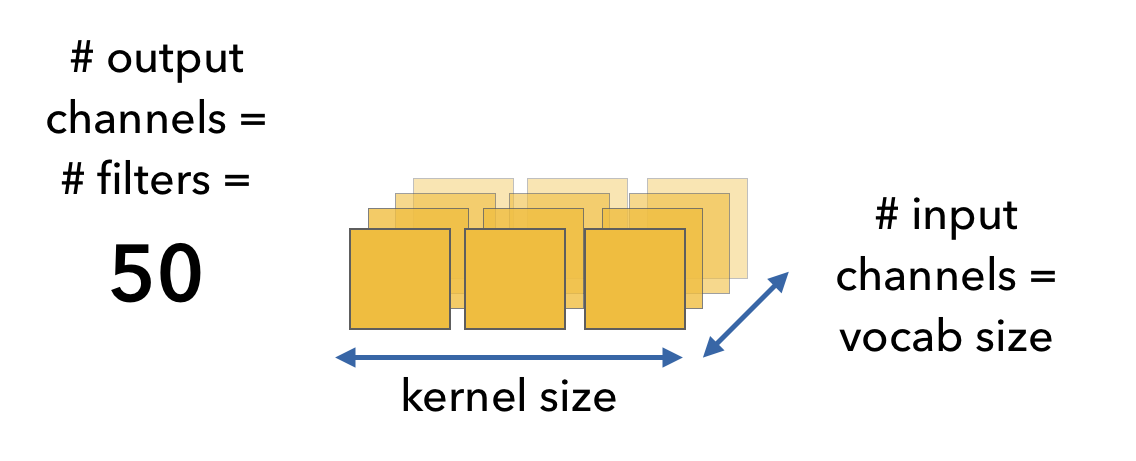• stride: amount the filters move from one convolution operation to the next.
• padding: values (typically zero) padded to the input, typically to create a volume with whole number dimensions.

So far we've used a stride of 1 and VALID padding (no padding) but let's look at an example with a higher stride and difference between different padding approaches.

• VALID: no padding, the filters only use the "valid" values in the input. If the filter cannot reach all the input values (filters go left to right), the extra values on the right are dropped.
• SAME: adds padding evenly to the right (preferred) and left sides of the input so that all values in the input are processed.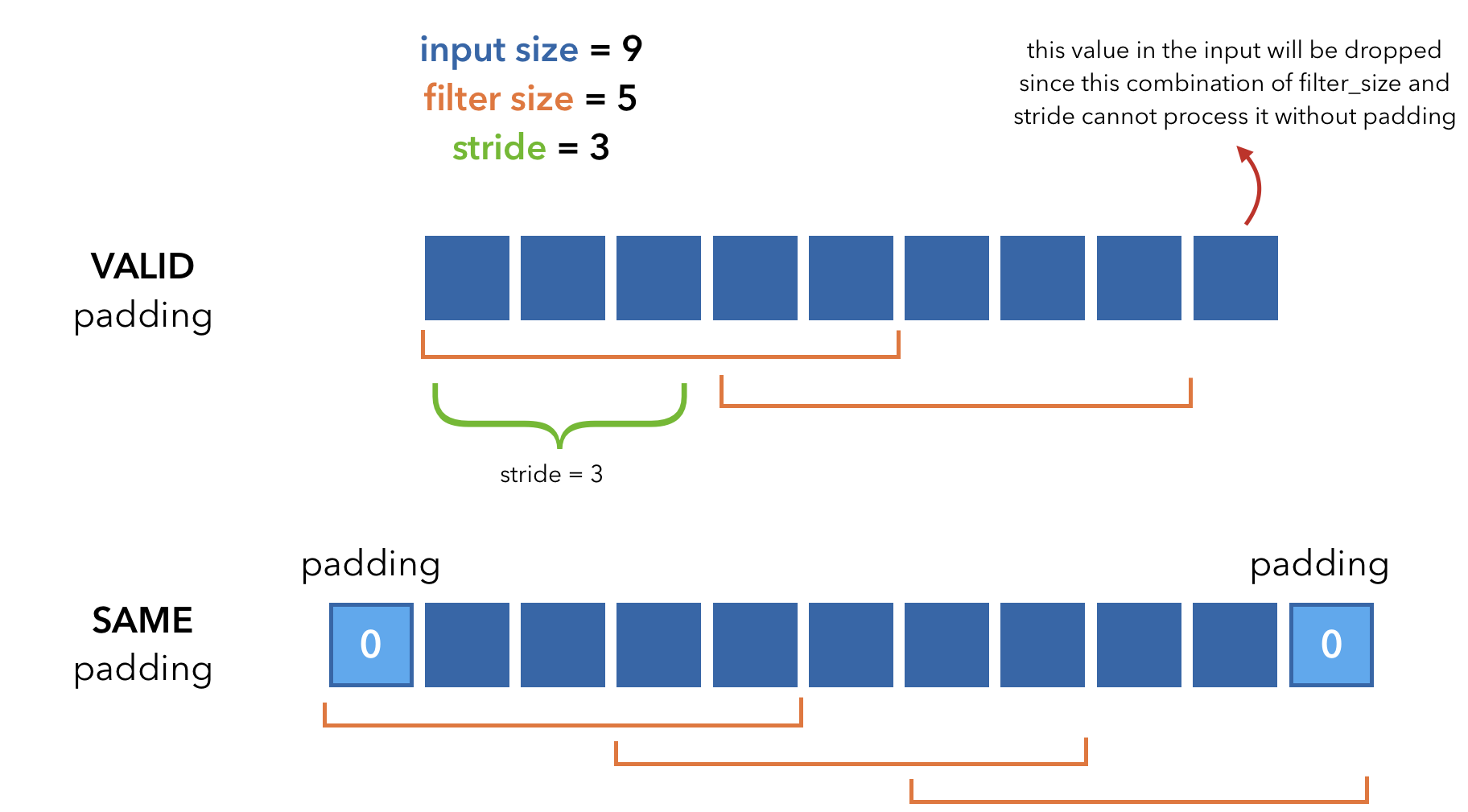We're going to use the Conv1d layer to process our inputs.

  1 2 3 4 5 6 7 8 9 10 # Convolutional filters (VALID padding) vocab_size = 10 # one hot size num_filters = 50 # num filters filter_size = 3 # filters are 3X3 stride = 1 padding = 0 # valid padding (no padding) conv1 = nn.Conv1d(in_channels=vocab_size, out_channels=num_filters, kernel_size=filter_size, stride=stride, padding=padding, padding_mode="zeros") print("conv: {}".format(conv1.weight.shape)) 
conv: torch.Size([50, 10, 3])

 1 2 3 # Forward pass z = conv1(x) print (f"z: {z.shape}") 
z: torch.Size([64, 50, 6])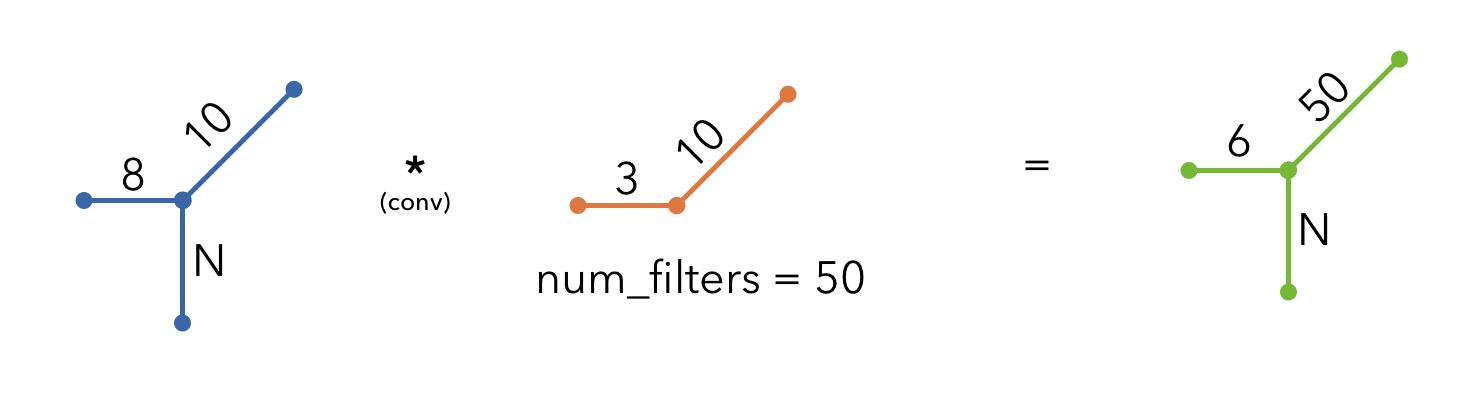When we apply these filter on our inputs, we receive an output of shape (N, 6, 50). We get 50 for the output channel dim because we used 50 filters and 6 for the conv outputs because:

$W_1 = \frac{W_2 - F + 2P}{S} + 1 = \frac{8 - 3 + 2(0)}{1} + 1 = 6$
$H_1 = \frac{H_2 - F + 2P}{S} + 1 = \frac{1 - 1 + 2(0)}{1} + 1 = 1$
$D_2 = D_1$

Variable Description
$$W$$ width of each input = 8
$$H$$ height of each input = 1
$$D$$ depth (# of channels)
$$F$$ filter size = 3
$$P$$ padding = 0
$$S$$ stride = 1

Now we'll add padding so that the convolutional outputs are the same shape as our inputs. The amount of padding for the SAME padding can be determined using the same equation. We want out output to have the same width as our input, so we solve for P:

$\frac{W-F+2P}{S} + 1 = W$
$P = \frac{S(W-1) - W + F}{2}$

If $$P$$ is not a whole number, we round up (using math.ceil) and place the extra padding on the right side.

 1 2 3 4 5 6 7 8 # Convolutional filters (SAME padding) vocab_size = 10 # one hot size num_filters = 50 # num filters filter_size = 3 # filters are 3X3 stride = 1 conv = nn.Conv1d(in_channels=vocab_size, out_channels=num_filters, kernel_size=filter_size, stride=stride) print("conv: {}".format(conv.weight.shape)) 
conv: torch.Size([50, 10, 3])

 1 2 3 4 # SAME padding padding_left = int((conv.stride*(max_seq_len-1) - max_seq_len + filter_size)/2) padding_right = int(math.ceil((conv.stride*(max_seq_len-1) - max_seq_len + filter_size)/2)) print (f"padding: {(padding_left, padding_right)}") 
padding: (1, 1)

 1 2 3 # Forward pass z = conv(F.pad(x, (padding_left, padding_right))) print (f"z: {z.shape}") 
z: torch.Size([64, 50, 8])


We will explore larger dimensional convolution layers in subsequent lessons. For example, Conv2D is used with 3D inputs (images, char-level text, etc.) and Conv3D is used for 4D inputs (videos, time-series, etc.).

### Pooling

The result of convolving filters on an input is a feature map. Due to the nature of convolution and overlaps, our feature map will have lots of redundant information. Pooling is a way to summarize a high-dimensional feature map into a lower dimensional one for simplified downstream computation. The pooling operation can be the max value, average, etc. in a certain receptive field. Below is an example of pooling where the outputs from a conv layer are 4X4 and we're going to apply max pool filters of size 2X2.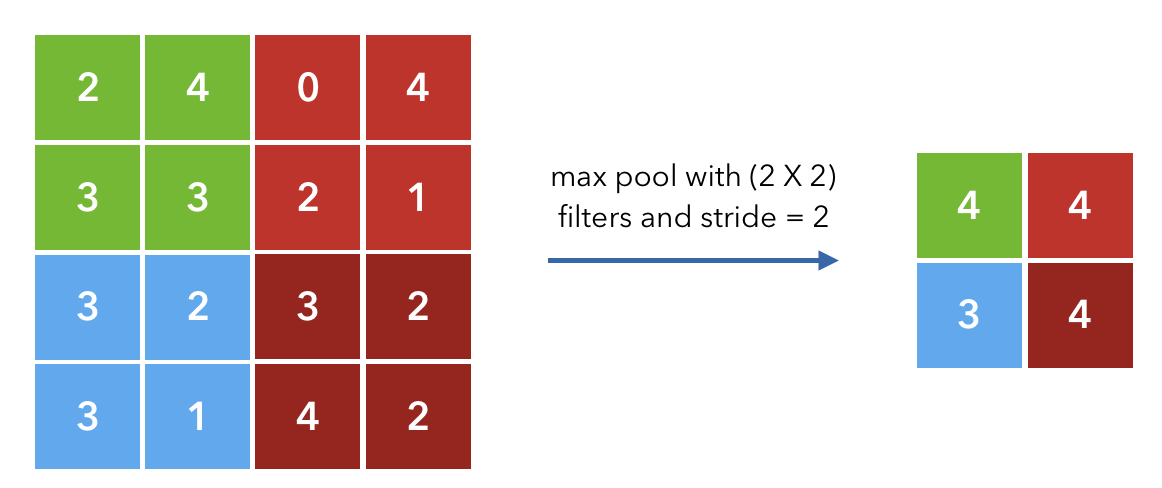$W_2 = \frac{W_1 - F}{S} + 1 = \frac{4 - 2}{2} + 1 = 2$
$H_2 = \frac{H_1 - F}{S} + 1 = \frac{4 - 2}{2} + 1 = 2$
$D_2 = D_1$

Variable Description
$$W$$ width of each input = 4
$$H$$ height of each input = 4
$$D$$ depth (# of channels)
$$F$$ filter size = 2
$$S$$ stride = 2

In our use case, we want to just take the one max value so we will use the MaxPool1D layer, so our max-pool filter size will be max_seq_len.

 1 2 3 # Max pooling pool_output = F.max_pool1d(z, z.size(2)) print("Size: {}".format(pool_output.shape)) 

Size: torch.Size([64, 50, 1])


### Batch normalization

The last topic we'll cover before constructing our model is batch normalization. It's an operation that will standardize (mean=0, std=1) the activations from the previous layer. Recall that we used to standardize our inputs in previous notebooks so our model can optimize quickly with larger learning rates. It's the same concept here but we continue to maintain standardized values throughout the repeated forward passes to further aid optimization.

 1 2 3 4 # Batch normalization batch_norm = nn.BatchNorm1d(num_features=num_filters) z = batch_norm(conv(x)) # applied to activations (after conv layer & before pooling) print (f"z: {z.shape}") 
z: torch.Size([64, 50, 6])

 1 2 # Mean and std before batchnorm print (f"mean: {torch.mean(conv(x)):.2f}, std: {torch.std(conv(x)):.2f}") 
mean: -0.00, std: 0.57

 1 2 # Mean and std after batchnorm print (f"mean: {torch.mean(z):.2f}, std: {torch.std(z):.2f}") 
mean: 0.00, std: 1.00


## Modeling

### Model

Let's visualize the model's forward pass.

1. We'll first tokenize our inputs (batch_size, max_seq_len).
2. Then we'll one-hot encode our tokenized inputs (batch_size, max_seq_len, vocab_size).
3. We'll apply convolution via filters (filter_size, vocab_size, num_filters) followed by batch normalization. Our filters act as character level n-gram detectors.
4. We'll apply 1D global max pooling which will extract the most relevant information from the feature maps for making the decision.
5. We feed the pool outputs to a fully-connected (FC) layer (with dropout).
6. We use one more FC layer with softmax to derive class probabilities.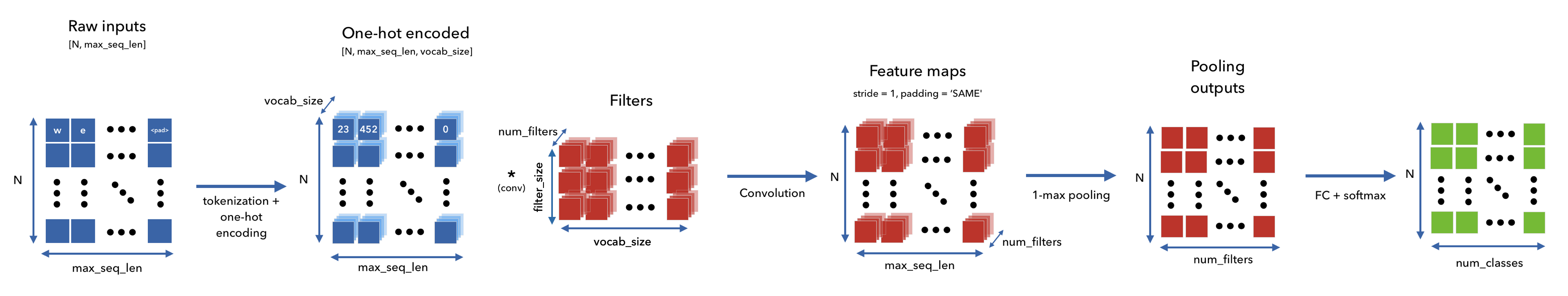1 2 3 NUM_FILTERS = 50 HIDDEN_DIM = 100 DROPOUT_P = 0.1 
  1 2 3 4 5 6 7 8 9 10 11 12 13 14 15 16 17 18 19 20 21 22 23 24 25 26 27 28 29 30 31 32 33 34 35 36 37 38 class CNN(nn.Module): def __init__(self, vocab_size, num_filters, filter_size, hidden_dim, dropout_p, num_classes): super(CNN, self).__init__() # Convolutional filters self.filter_size = filter_size self.conv = nn.Conv1d( in_channels=vocab_size, out_channels=num_filters, kernel_size=filter_size, stride=1, padding=0, padding_mode="zeros") self.batch_norm = nn.BatchNorm1d(num_features=num_filters) # FC layers self.fc1 = nn.Linear(num_filters, hidden_dim) self.dropout = nn.Dropout(dropout_p) self.fc2 = nn.Linear(hidden_dim, num_classes) def forward(self, inputs, channel_first=False,): # Rearrange input so num_channels is in dim 1 (N, C, L) x_in, = inputs if not channel_first: x_in = x_in.transpose(1, 2) # Padding for SAME padding max_seq_len = x_in.shape padding_left = int((self.conv.stride*(max_seq_len-1) - max_seq_len + self.filter_size)/2) padding_right = int(math.ceil((self.conv.stride*(max_seq_len-1) - max_seq_len + self.filter_size)/2)) # Conv outputs z = self.conv(F.pad(x_in, (padding_left, padding_right))) z = F.max_pool1d(z, z.size(2)).squeeze(2) # FC layer z = self.fc1(z) z = self.dropout(z) z = self.fc2(z) return z 
 1 2 3 4 5 # Initialize model model = CNN(vocab_size=VOCAB_SIZE, num_filters=NUM_FILTERS, filter_size=FILTER_SIZE, hidden_dim=HIDDEN_DIM, dropout_p=DROPOUT_P, num_classes=NUM_CLASSES) model = model.to(device) # set device print (model.named_parameters) 

<bound method Module.named_parameters of CNN(
(conv): Conv1d(500, 50, kernel_size=(1,), stride=(1,))
(batch_norm): BatchNorm1d(50, eps=1e-05, momentum=0.1, affine=True, track_running_stats=True)
(fc1): Linear(in_features=50, out_features=100, bias=True)
(dropout): Dropout(p=0.1, inplace=False)
(fc2): Linear(in_features=100, out_features=4, bias=True)
)>


We used SAME padding (w/ stride=1) which means that the conv outputs will have the same width (max_seq_len) as our inputs. The amount of padding differs for each batch based on the max_seq_len but you can calculate it by solving for P in the equation below.

$\frac{W_1 - F + 2P}{S} + 1 = W_2$
$\frac{\text{max_seq_len } - \text{ filter_size } + 2P}{\text{stride}} + 1 = \text{max_seq_len}$
$P = \frac{\text{stride}(\text{max_seq_len}-1) - \text{max_seq_len} + \text{filter_size}}{2}$

If $$P$$ is not a whole number, we round up (using math.ceil) and place the extra padding on the right side.

### Training

Let's create the Trainer class that we'll use to facilitate training for our experiments. Notice that we're now moving the train function inside this class.

 1 from torch.optim import Adam 
 1 2 3 LEARNING_RATE = 1e-3 PATIENCE = 5 NUM_EPOCHS = 10 
  1 2 3 4 5 6 7 8 9 10 11 12 13 14 15 16 17 18 19 20 21 22 23 24 25 26 27 28 29 30 31 32 33 34 35 36 37 38 39 40 41 42 43 44 45 46 47 48 49 50 51 52 53 54 55 56 57 58 59 60 61 62 63 64 65 66 67 68 69 70 71 72 73 74 75 76 77 78 79 80 81 82 83 84 85 86 87 88 89 90 91 92 93 94 95 96 97 98 99 100 101 102 103 104 105 106 107 108 class Trainer(object): def __init__(self, model, device, loss_fn=None, optimizer=None, scheduler=None): # Set params self.model = model self.device = device self.loss_fn = loss_fn self.optimizer = optimizer self.scheduler = scheduler def train_step(self, dataloader): """Train step.""" # Set model to train mode self.model.train() loss = 0.0 # Iterate over train batches for i, batch in enumerate(dataloader): # Step batch = [item.to(self.device) for item in batch] # Set device inputs, targets = batch[:-1], batch[-1] self.optimizer.zero_grad() # Reset gradients z = self.model(inputs) # Forward pass J = self.loss_fn(z, targets) # Define loss J.backward() # Backward pass self.optimizer.step() # Update weights # Cumulative Metrics loss += (J.detach().item() - loss) / (i + 1) return loss def eval_step(self, dataloader): """Validation or test step.""" # Set model to eval mode self.model.eval() loss = 0.0 y_trues, y_probs = [], [] # Iterate over val batches with torch.inference_mode(): for i, batch in enumerate(dataloader): # Step batch = [item.to(self.device) for item in batch] # Set device inputs, y_true = batch[:-1], batch[-1] z = self.model(inputs) # Forward pass J = self.loss_fn(z, y_true).item() # Cumulative Metrics loss += (J - loss) / (i + 1) # Store outputs y_prob = F.softmax(z).cpu().numpy() y_probs.extend(y_prob) y_trues.extend(y_true.cpu().numpy()) return loss, np.vstack(y_trues), np.vstack(y_probs) def predict_step(self, dataloader): """Prediction step.""" # Set model to eval mode self.model.eval() y_probs = [] # Iterate over val batches with torch.inference_mode(): for i, batch in enumerate(dataloader): # Forward pass w/ inputs inputs, targets = batch[:-1], batch[-1] z = self.model(inputs) # Store outputs y_prob = F.softmax(z).cpu().numpy() y_probs.extend(y_prob) return np.vstack(y_probs) def train(self, num_epochs, patience, train_dataloader, val_dataloader): best_val_loss = np.inf for epoch in range(num_epochs): # Steps train_loss = self.train_step(dataloader=train_dataloader) val_loss, _, _ = self.eval_step(dataloader=val_dataloader) self.scheduler.step(val_loss) # Early stopping if val_loss < best_val_loss: best_val_loss = val_loss best_model = self.model _patience = patience # reset _patience else: _patience -= 1 if not _patience: # 0 print("Stopping early!") break # Logging print( f"Epoch: {epoch+1} | " f"train_loss: {train_loss:.5f}, " f"val_loss: {val_loss:.5f}, " f"lr: {self.optimizer.param_groups['lr']:.2E}, " f"_patience: {_patience}" ) return best_model 
 1 2 3 # Define Loss class_weights_tensor = torch.Tensor(list(class_weights.values())).to(device) loss_fn = nn.CrossEntropyLoss(weight=class_weights_tensor) 
 1 2 3 4 # Define optimizer & scheduler optimizer = Adam(model.parameters(), lr=LEARNING_RATE) scheduler = torch.optim.lr_scheduler.ReduceLROnPlateau( optimizer, mode="min", factor=0.1, patience=3) 
 1 2 3 4 # Trainer module trainer = Trainer( model=model, device=device, loss_fn=loss_fn, optimizer=optimizer, scheduler=scheduler) 
 1 2 3 # Train best_model = trainer.train( NUM_EPOCHS, PATIENCE, train_dataloader, val_dataloader) 

Epoch: 1 | train_loss: 0.87388, val_loss: 0.79013, lr: 1.00E-03, _patience: 3
Epoch: 2 | train_loss: 0.78354, val_loss: 0.78657, lr: 1.00E-03, _patience: 3
Epoch: 3 | train_loss: 0.77743, val_loss: 0.78433, lr: 1.00E-03, _patience: 3
Epoch: 4 | train_loss: 0.77242, val_loss: 0.78260, lr: 1.00E-03, _patience: 3
Epoch: 5 | train_loss: 0.76900, val_loss: 0.78169, lr: 1.00E-03, _patience: 3
Epoch: 6 | train_loss: 0.76613, val_loss: 0.78064, lr: 1.00E-03, _patience: 3
Epoch: 7 | train_loss: 0.76413, val_loss: 0.78019, lr: 1.00E-03, _patience: 3
Epoch: 8 | train_loss: 0.76215, val_loss: 0.78016, lr: 1.00E-03, _patience: 3
Epoch: 9 | train_loss: 0.76034, val_loss: 0.77974, lr: 1.00E-03, _patience: 3
Epoch: 10 | train_loss: 0.75859, val_loss: 0.77978, lr: 1.00E-03, _patience: 2


### Evaluation

 1 2 3 import json from pathlib import Path from sklearn.metrics import precision_recall_fscore_support 
  1 2 3 4 5 6 7 8 9 10 11 12 13 14 15 16 17 18 19 20 21 22 23 def get_metrics(y_true, y_pred, classes): """Per-class performance metrics.""" # Performance performance = {"overall": {}, "class": {}} # Overall performance metrics = precision_recall_fscore_support(y_true, y_pred, average="weighted") performance["overall"]["precision"] = metrics performance["overall"]["recall"] = metrics performance["overall"]["f1"] = metrics performance["overall"]["num_samples"] = np.float64(len(y_true)) # Per-class performance metrics = precision_recall_fscore_support(y_true, y_pred, average=None) for i in range(len(classes)): performance["class"][classes[i]] = { "precision": metrics[i], "recall": metrics[i], "f1": metrics[i], "num_samples": np.float64(metrics[i]), } return performance 
 1 2 3 # Get predictions test_loss, y_true, y_prob = trainer.eval_step(dataloader=test_dataloader) y_pred = np.argmax(y_prob, axis=1) 
 1 2 3 4 # Determine performance performance = get_metrics( y_true=y_test, y_pred=y_pred, classes=label_encoder.classes) print (json.dumps(performance["overall"], indent=2)) 

{
"precision": 0.7120047175492572,
"recall": 0.6935,
"f1": 0.6931471439737603,
"num_samples": 18000.0
}

 1 2 3 4 5 6 7 8 # Save artifacts dir = Path("cnn") dir.mkdir(parents=True, exist_ok=True) label_encoder.save(fp=Path(dir, "label_encoder.json")) tokenizer.save(fp=Path(dir, 'tokenizer.json')) torch.save(best_model.state_dict(), Path(dir, "model.pt")) with open(Path(dir, 'performance.json'), "w") as fp: json.dump(performance, indent=2, sort_keys=False, fp=fp) 

### Inference

 1 2 3 4 5 6 7 8 def get_probability_distribution(y_prob, classes): """Create a dict of class probabilities from an array.""" results = {} for i, class_ in enumerate(classes): results[class_] = np.float64(y_prob[i]) sorted_results = {k: v for k, v in sorted( results.items(), key=lambda item: item, reverse=True)} return sorted_results 
 1 2 3 4 5 6 7 8 9 # Load artifacts device = torch.device("cpu") label_encoder = LabelEncoder.load(fp=Path(dir, "label_encoder.json")) tokenizer = Tokenizer.load(fp=Path(dir, 'tokenizer.json')) model = CNN( vocab_size=VOCAB_SIZE, num_filters=NUM_FILTERS, filter_size=FILTER_SIZE, hidden_dim=HIDDEN_DIM, dropout_p=DROPOUT_P, num_classes=NUM_CLASSES) model.load_state_dict(torch.load(Path(dir, "model.pt"), map_location=device)) model.to(device) 

CNN(
(conv): Conv1d(500, 50, kernel_size=(1,), stride=(1,))
(batch_norm): BatchNorm1d(50, eps=1e-05, momentum=0.1, affine=True, track_running_stats=True)
(fc1): Linear(in_features=50, out_features=100, bias=True)
(dropout): Dropout(p=0.1, inplace=False)
(fc2): Linear(in_features=100, out_features=4, bias=True)
)


 1 2 # Initialize trainer trainer = Trainer(model=model, device=device) 
 1 2 3 4 5 6 7 8 # Dataloader text = "What a day for the new york stock market to go bust!" sequences = tokenizer.texts_to_sequences([preprocess(text)]) print (tokenizer.sequences_to_texts(sequences)) X = [to_categorical(seq, num_classes=len(tokenizer)) for seq in sequences] y_filler = label_encoder.encode([label_encoder.classes]*len(X)) dataset = Dataset(X=X, y=y_filler, max_filter_size=FILTER_SIZE) dataloader = dataset.create_dataloader(batch_size=batch_size) 

['day new <UNK> stock market go <UNK>']

 1 2 3 4 # Inference y_prob = trainer.predict_step(dataloader) y_pred = np.argmax(y_prob, axis=1) label_encoder.decode(y_pred) 
['Business']

 1 2 3 # Class distributions prob_dist = get_probability_distribution(y_prob=y_prob, classes=label_encoder.classes) print (json.dumps(prob_dist, indent=2)) 
{
"Sci/Tech": 0.10699427127838135,
"World": 0.021050667390227318,
"Sports": 0.004871787969022989
}


## Interpretability

We went through all the trouble of padding our inputs before convolution to result in outputs of the same shape as our inputs so we can try to get some interpretability. Since every token is mapped to a convolutional output on which we apply max pooling, we can see which token's output was most influential towards the prediction. We first need to get the conv outputs from our model:

 1 2 import collections import seaborn as sns 
  1 2 3 4 5 6 7 8 9 10 11 12 13 14 15 16 17 18 19 20 21 22 23 24 25 26 27 28 29 30 31 32 class InterpretableCNN(nn.Module): def __init__(self, vocab_size, num_filters, filter_size, hidden_dim, dropout_p, num_classes): super(InterpretableCNN, self).__init__() # Convolutional filters self.filter_size = filter_size self.conv = nn.Conv1d( in_channels=vocab_size, out_channels=num_filters, kernel_size=filter_size, stride=1, padding=0, padding_mode="zeros") self.batch_norm = nn.BatchNorm1d(num_features=num_filters) # FC layers self.fc1 = nn.Linear(num_filters, hidden_dim) self.dropout = nn.Dropout(dropout_p) self.fc2 = nn.Linear(hidden_dim, num_classes) def forward(self, inputs, channel_first=False): # Rearrange input so num_channels is in dim 1 (N, C, L) x_in, = inputs if not channel_first: x_in = x_in.transpose(1, 2) # Padding for SAME padding max_seq_len = x_in.shape padding_left = int((self.conv.stride*(max_seq_len-1) - max_seq_len + self.filter_size)/2) padding_right = int(math.ceil((self.conv.stride*(max_seq_len-1) - max_seq_len + self.filter_size)/2)) # Conv outputs z = self.conv(F.pad(x_in, (padding_left, padding_right))) return z 
 1 2 3 4 # Initialize interpretable_model = InterpretableCNN( vocab_size=len(tokenizer), num_filters=NUM_FILTERS, filter_size=FILTER_SIZE, hidden_dim=HIDDEN_DIM, dropout_p=DROPOUT_P, num_classes=NUM_CLASSES) 
 1 2 3 # Load weights (same architecture) interpretable_model.load_state_dict(torch.load(Path(dir, "model.pt"), map_location=device)) interpretable_model.to(device) 

InterpretableCNN(
(conv): Conv1d(500, 50, kernel_size=(1,), stride=(1,))
(batch_norm): BatchNorm1d(50, eps=1e-05, momentum=0.1, affine=True, track_running_stats=True)
(fc1): Linear(in_features=50, out_features=100, bias=True)
(dropout): Dropout(p=0.1, inplace=False)
(fc2): Linear(in_features=100, out_features=4, bias=True)
)


 1 2 # Initialize trainer interpretable_trainer = Trainer(model=interpretable_model, device=device) 
 1 2 3 # Get conv outputs conv_outputs = interpretable_trainer.predict_step(dataloader) print (conv_outputs.shape) # (num_filters, max_seq_len) 

(50, 7)

 1 2 3 # Visualize a bi-gram filter's outputs tokens = tokenizer.sequences_to_texts(sequences).split(" ") sns.heatmap(conv_outputs, xticklabels=tokens)The filters have high values for the words stock and market which influenced the Business category classification.

Warning

This is a crude technique loosely based off of more elaborate interpretability methods.

To cite this content, please use:

 1 2 3 4 5 6 @article{madewithml, author = {Goku Mohandas}, title = { CNNs - Made With ML }, howpublished = {\url{https://madewithml.com/}}, year = {2023} }